# Viscoelastic Mechanical Model of Asphalt Concrete Considering the Influence of Characteristic Parameter of Fiber Content

Viscoelastic Mechanical Model of Asphalt Concrete Considering the Influence of Characteristic Parameter of Fiber Content
College of Civil Engineering, Xuchang University, Xuchang 461000, China

Zhengzhou University, Zhengzhou 450000, China

Henan University of Urban Construction, Pingdingshan 467000, China

Corresponding Author Email:
chunshuihuang@163.com
Page:
283-290
|
DOI:
https://doi.org/10.18280/rcma.310503
5 July 2021
|
Accepted:
12 September 2021
|
Published:
31 October 2021
| Citation

OPEN ACCESS

Abstract:

This paper carries out bending creep tests on polyester fiber-reinforced asphalt concrete beams, and investigates how the volume ratio and aspect ratio of the fibers influence the parameters of viscoelastic mechanical model and the viscoelastic performance of the asphalt concrete. The results show that: with the growing volume ratio and aspect ratio of the fibers, the midspan deflection and bottom flexural-tensile strain of asphalt concrete beams first dropped and then rose over time; the characteristic parameter of fiber content (FCCP) could reflect the overall effects of the volume ratio and aspect ratio of the fibers. On this basis, a viscoelastic mechanical model was established for the asphalt concrete in the light of the influence of FCCP. The test and theoretical results show that, in our tests, the optimal volume ratio of fibers, optimal aspect ratio of fibers, and optimal FCCP are 0.348, 324, and 1.128 for polyester fiber-reinforced asphalt concrete.

Keywords:

fiber-reinforced asphalt concrete, viscoelastic performance, bending creep test, characteristic parameter of fiber content (FCCP)

1. Introduction

Asphalt concrete is a typical viscoelastic composite. The influence of temperature and load stress on the viscoelastic properties of asphalt concrete is mainly investigated with different viscoelastic mechanical models composed of basic viscoelastic components (e.g., springs and dampers), based on uniaxial compressive creep, triaxial compressive creep, and beam bending creep tests [1-10].

Since the addition of fibers can significantly improve the road performance of asphalt concrete, more and more attention has been paid to the viscoelastic properties of fiber-reinforced asphalt concrete. Through dynamic and static triaxial tests, Benedito et al.  studied the influence of fiber dosage and length on cold-mix asphalt concrete. Guo et al.  and Guo et al.  conducted compressive creep tests on polyester fiber-reinforced asphalt concrete, and adopted Burgers model and modified Burgers model to examine the influence of fibers of the same length over the viscoelastic performance of asphalt concrete at different dosages. On this basis, Guo established a viscoelastic mechanical model of asphalt concrete considering the influence of fiber dosage. Feng  analyzed the effects of fiber length on the performance of asphalt concrete through a pull-out test.

However, many research problems are yet to be solved: How does the aspect ratio of fibers impact the parameters of viscoelastic mechanical model and the viscoelastic performance of asphalt concrete? How do the volume ratio (or dosage) and aspect ratio of fibers affect the viscoelastic mechanical model and the viscoelastic performance of asphalt concrete after unloading? What is the composite influence of the volume ratio and aspect ratio of fibers over the viscoelastic performance of asphalt concrete?

To answer these questions, this paper takes the AC-13F asphalt mixture as the matrix, adopts the volume ratio Vf and aspect ratio Ra as parameters, and tests the bending creep of polyester fiber-reinforced asphalt concrete beams, based on the optimal asphalt dosage determined by Marshall test. Next, Burgers model and modified Burgers model were employed to systematically analyze the influence of Vf and Ra over the parameters of viscoelastic mechanical model and the viscoelastic performance of the asphalt concrete during the loading and unloading phases. After that, the composite influence of the two parameters was characterized by the characteristic parameter of fiber content (FCCP) λf, and a viscoelastic mechanical model was established for the asphalt concrete considering the influence of λf.

2. Methodology

Our tests use petroleum asphalt 70 and polyester fibers with a mean diameter of 18.5μm. To study the influence of the volume ratio Vf and aspect ratio Ra of the fibers, the fiber length was set to 3mm, 6mm, and 9mm, respectively; the aspect ratio was set to 162, 324, 486, and 649, respectively. For the polyester fibers of the length 3mm, 9mm, and 12mm, the volume ratio was set to 0.351, 0.34, and 0.346 in turn. For those of the length 6mm, the volume ratio was set to 0.174, 0.348, 0.519, and 0.686 in turn.

The aggregates were screened, cleaned, and dried, and then mixed with limestone powder back to AC-13 asphalt concrete with medium grading. Through standard Marshall test , the optimal asphalt content (OAC) was determined for the matrix of asphalt mix, and for the asphalt mix with each volume ratio and aspect ratio of the fibers.

The designed polyester fiber-reinforced asphalt mix was compressed into specimens of 300mm×300mm×50mm, and then cut into small beams of 250mm×30mm×35mm. Then, creep tests were carried out at 15℃ on a multi-function material testing machine. The specimens were loaded with weights. The test environment is illustrated in Figure 1. The creep load is 10% of the bending failure load of the beam under the same conditions.

## 1.jpg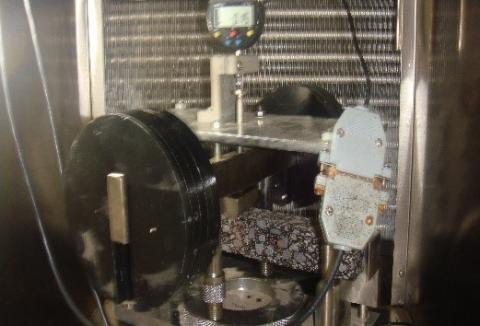Figure 1. Creep test environment

The creep tests were performed on eight groups of small beams. Each group contains three small beams. During the tests, the midspan deflection d(t) of the small beam being tested was collected by a dial gauge, which is connected to the data acquisition system, and the time-deflection curve was plotted. When the small beam entered accelerated creep phase, the load was removed, and the midspan deflection was collected 30min after the unloading. Based on the midspan deflection, the bottom flexural-tensile strain can be calculated by:

$\varepsilon (t)=\frac{6hd(t)}{{{L}^{2}}}$            (1)

where, h is the height of the specimen (m); L is the span of the specimen (m).

3. Viscoelastic Deformation Features

Figures 2 and 3 show the time variation of midspan deflection and bottom flexural-tensile strain of the small asphalt concrete beams of different fiber volume ratios and fiber aspect ratios, respectively. It can be observed that, with the growing volume ratio and aspect ratio of the fibers, the midspan deflection and bottom flexural-tensile strain of asphalt concrete beams first dropped and then rose over time, exhibiting a nonlinear trend. The lowest point of the time-creep deformation curve appeared at the fiber volume ratio of 0.348 and the fiber aspect ratio of 324.

## 2.png(a) Mid-span deflection

## 2b.png(b) Strain

Figure 2. Relationship between creep deformation and fiber volume ratio

## 3a.png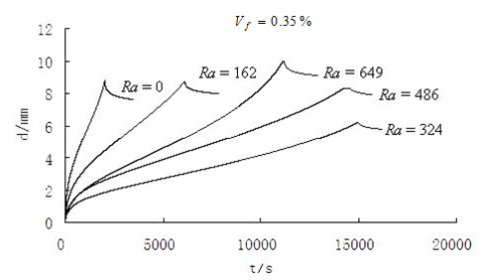(a) Mid-span deflection

## 3b.png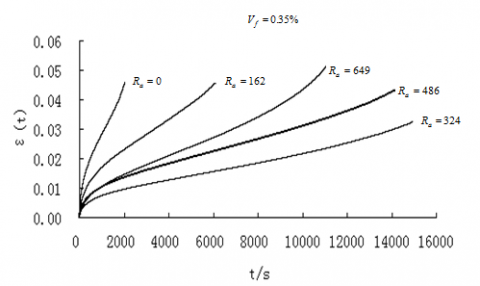(b) Strain

Figure 3. Relationship between creep deformation and fiber aspect ratio

The reason is that, when the fiber aspect ratio is fixed, the three-dimensional (3D) fiber mesh in the asphalt concrete becomes denser, and the fibers can better constrain the deformation of asphalt concrete. When the fiber volume ratio surpasses 0.348, the fibers are less dispersive, because the fiber mesh is too dense. The undispersed fibers will cluster into bundles, forming defects in the asphalt concrete . In this case, the fibers will reduce the viscoelastic performance of asphalt concrete, rather than enhance the viscoelasticity. When the fiber volume ratio remains constant, a high fiber aspect ratio means the soft fibers are long, with poor directionality, and easily curled and caked; a small fiber aspect ratio means there are many fibers per unit volume of asphalt concrete, i.e., the fiber mesh is so dense that the fibers are prone to caking, which weakens the reinforcement effect of fibers.

The lower the time-creep deformation curve, the stronger the asphalt concrete against rutting deformation . In our tests, the fiber-reinforced asphalt concrete can resist rutting deformation excellently at the fiber volume ratio of 0.348 and the fiber aspect ratio of 324.

It can also be seen from Figures 2 and 3 that, although the fiber-reinforced asphalt concretes with different fiber volume ratios and fiber aspect ratios differed in creep deformation, their time-creep deformation curves all consist of three segments: decelerated creep, constant speed creep, and accelerated creep. The addition of fibers does not change the creep features of the asphalt concrete. Therefore, it is possible to derive a viscoelastic mechanical model for fiber-reinforced asphalt concrete from the viscoelastic mechanical model for ordinary asphalt concrete, by incorporating the fiber influence in model parameters.

4. Viscoelastic Mechanical Model

Referring to the Burgers model [7, 13] in Figure 4, the viscoelastic creep equation of the fiber-reinforced asphalt concrete can be expressed as:

$\varepsilon=\sigma_{0}\left(\frac{1}{\mathrm{E}_{1}}+\frac{t}{\eta_{1}}+\frac{1-e^{-t \tau}}{\mathrm{E}_{2}}\right)$       (2)

$\varepsilon=\sigma_{0}\left[\frac{t_{0}}{\eta_{1}}+\frac{\left(1-e^{-t_{0} \tau}\right) e^{-\tau\left(t-t_{0}\right)\quad}}{\mathrm{E}_{2}}\right]$               (3)

where, Ε1 and η1 are the elastic modulus of elastic element 1 and viscosity of viscous element 4 considering fiber influence, respectively; Ε2 and η2 are the elastic modulus of elastic element 2 and viscosity of viscous element 3 considering fiber influence, respectively; $\tau=\frac{E_{2}}{\eta_{2}}$.

## 4.pngFigure 4. Burgers model

## 5.pngFigure 5. Modified Burgers model

The greater the value of Ε1, the stronger the elastic deformation resistance of the asphalt concrete; the greater the value of η1, the smaller the permanent deformation induced by the same load in the same period; the greater the value of η2/Ε2 the slower the growth of viscous deformation over time, and the shallower the rutting depth on the asphalt concrete pavement; the greater the value of η2/Ε2, the slower the development of asphalt concrete deformation, and the stronger the resistance to viscoelastic deformation .

Drawing on the modified Burgers model (Figure 5) , the viscoelastic creep equation of fiber-reinforced asphalt concrete can be expressed as:

$\varepsilon(t)=\sigma_{0}\left(\frac{1}{\mathrm{E}_{1}}+\frac{1-e^{-B t}}{A B}+\frac{1-e^{-t \tau}}{\mathrm{E}_{2}}\right)$              (4)

$\varepsilon (t)={{\sigma }_{0}}\left[ \frac{1-{{e}^{-B{{t}_{0}}}}}{AB}+\frac{(1-{{e}^{-{{t}_{0}}\tau }}){{e}^{-(t-{{t}_{0}})\tau\quad }}}{{{E}_{2}}} \right]$               (5)

where, σ0 is the test stress; t is the test time; t0 is the total loading time.

Based on the creep test results, the parameters of the viscoelastic mechanical model (2)-(5) were initialized through the nonlinear fitting approach of Origin 8.5. By adjusting model parameters, the fitting approach that maximize the matching between theoretical results and the test data was adopted to determine the parameters of the viscoelastic mechanical model. On this basis, the authors analyzed the influence of fiber volume ratio and fiber aspect ratio on model parameters. Then, the FCCP was introduced to reflect the combined effect of fiber volume ratio and fiber aspect ratio, and used to build the constitutive equation of the fiber-reinforced asphalt concrete. Finally, the viscoelasticity of the fiber-reinforced asphalt concrete was examined by the creep equation and the constitutive equation.

4.1 Influence of fiber volume ratio and fiber aspect ratio on viscoelastic model parameters and viscoelastic performance

Table 1 shows the viscoelastic model parameters of the fiber-reinforced asphalt concrete with different fiber volume ratios and fiber aspect ratios, which are fitted by formula (2). It can be observed that Ε1 first increased and then decreased, with the growing fiber volume ratio. The peak of Ε1 appeared at the fiber volume ratio of 0.348. In this case, the fiber-reinforced asphalt concrete has a strong resistance to the elastic deformation under instantaneous load. When the fiber volume ratio reached 0.686, the Ε1 of the fiber-reinforced asphalt concrete was smaller than that of the asphalt concrete in the matrix, indicating that the fibers can enhance the elasticity of the fiber-reinforced asphalt concrete only under a proper volume ratio.

With the increase of fiber volume ratio, the η1 first increased and then declined, suggesting the good thickening effect of a proper dosage of fibers. When the fiber volume ratio was 0.348, the η1 more than doubled from that of the asphalt concrete in the matrix. In this case, the fibers exhibit a good thickening effect, and the fiber-reinforced asphalt concrete is good at resisting permanent deformation. After the fiber volume ratio reached 0.686, the fiber-reinforced asphalt concrete had a smaller η1 than the asphalt concrete in the matrix, that is, an excessive number of fibers would weaken the ability of the asphalt concrete to withstand permanent deformation.

Table 1. Relationship between parameters of formula (2) and fiber volume ratio and fiber aspect ratio

 Vf Ra Model parameters R2 Relaxation time/s Delay time/s Ε1/MPa Ε2/MPa η1/MPa·s η2/MPa·s 0 0 629 110 305403 30110 0.99912 485 274 0.174 324 726 144 530628 43517 0.99815 731 302 0.348 324 916 161 683955 51518 0.99540 746 320 0.519 324 793 148 501683 46680 0.99923 633 315 0.686 324 610 122 268829 36772 0.99913 315 301 0.351 162 823 144 541533 43359 0.99936 658 301 0.347 486 705 139 364537 43405 0.99746 517 312 0.346 649 643 125 319489 37890 0.99118 497 303

Table 2. Relationship between parameters of formula (3) and fiber volume ratio and fiber aspect ratio

 Vf Ra Model parameters R2 Delay time/s Ε2/MPa η1/MPa·s η2/MPa·s 0 0 343 251554 109199 0.98485 318 0.174 324 386 346085 145944 0.97533 378 0.348 324 406 399097 170332 0.99189 420 0.519 324 379 317203 140118 0.98291 370 0.686 324 345 184746 119707 0.98901 347 0.351 162 369 310862 141021 0.98455 382 0.347 486 364 292954 139592 0.99533 383 0.346 649 349 266937 123008 0.98254 352

The relaxation time η1/Ε1 and delay time η2/Ε2 of the fiber-reinforced asphalt concrete both increased and then decreased with the growth of fiber volume ratio. Therefore, a proper dosage of fibers could enhance the resistance of the asphalt concrete to rutting deformation. The relaxation time and delay time reached the peak at the fiber volume ratio of 0.348. In this case, the fibers significantly enhance the resistance of the asphalt concrete to rutting deformation .

Ε1, η1, η1/Ε1 and η2/Ε2 all increased first and then dropped with the growth of fiber aspect ratio, and peaked at the fiber aspect ratio of 324. In this case, the fiber-reinforced asphalt concrete boasts a strong capability to resist elastic deformation, viscous flow deformation, and rutting deformation.

Table 3 shows the viscoelastic model parameters of the fiber-reinforced asphalt concrete with different fiber volume ratios and fiber aspect ratios, which are fitted by formula (3). It can be observed that, Ε2 and η2 were much greater than those in the creep loading phase; the delay time η2/Ε2 was longer than that in the creep loading phase; η1 was much smaller than that in that phase.

Ε2, η1 and η2 all increased first and then decreased with the growth of fiber volume ratio and fiber aspect ratio. The peak values were observed at the fiber volume ratio of 0.348 and the fiber aspect ratio of 324. The variation law was the same as that in the creep loading phase.

It can also be seen from Table 2 that the correlation between test data and formula (3) in the creep unloading phase was weaker than that in the creep loading phase. Overall, formulas (2) and (3) can characterize the viscoelastic deformation features of asphalt concrete excellently during the loading phase.

Table 3 shows the viscoelastic model parameters of the fiber-reinforced asphalt concrete with different fiber volume ratios and fiber aspect ratios, which are fitted by formula (4). It can be observed that formula (4) is more closely correlated with the test data in the creep loading phase than formula (2). The parameters of formula (4), namely, Ε1, Ε2, η2, A and B all first increased and then decreased with the growth of fiber volume ratio and fiber aspect ratio. The peak values were observed at the fiber volume ratio of 0.348 and the fiber aspect ratio of 324. In this case, the fiber-reinforced asphalt concrete boasts a strong capability to resist elastic deformation, viscous flow deformation, and rutting deformation.

Table 4 shows the viscoelastic model parameters of the fiber-reinforced asphalt concrete with different fiber volume ratios and fiber aspect ratios, which are fitted by formula (5). It can be observed that the parameters of formula (5), namely, Ε2, η2, A and B all first increased and then decreased with the growth of fiber volume ratio and fiber aspect ratio. The peak values were observed at the fiber volume ratio of 0.348 and the fiber aspect ratio of 324. The change law was the same as that in formula (4). The only difference between formulas (5) and (4) lies in the variation of the value of the same parameter.

Compared with those in the creep loading phase, the model parameters Ε2 and B were relatively large, and η2 and A were relatively small in the creep unloading phase. This phenomenon indicates that the asphalt concrete recovers slower from viscoelastic deformation, and deforms more significantly than the theoretical permanent deformation, after being damaged in creep loading. Although formulas (4) and (5) describe different creep phases from formulas (2) and (3), the changes of their parameters demonstrate the same influence law of creep loading damages on material performance.

It can also be learned from Table 4 that formula (5) is much less correlated with the test data in the creep unloading phase than formula (4), and slightly less correlated with the test data in that phase than formula (3). Hence, formulas (4) and (5) are not as good as formulas (2) and (3) in characterizing the deformation features of unloaded asphalt concrete. To sum up, formulas (4) and (5) work well in characterizing the deformation features of the asphalt concrete and fiber-reinforced asphalt concrete in the loading phase, while formulas (2) and (3) work well in characterizing the deformation features of the asphalt concrete and fiber-reinforced asphalt concrete in the unloading phase.

Table 3. Relationship between parameters of formula (4) and fiber volume ratio and fiber aspect ratio

 Vf Ra Model parameters R2 Delay time/s Ε1/MPa Ε2/MPa η2/MPa·s A/MPa B/MPa 0 0 492 112 339492 38117 0.000200 0.9993 3031 0.174 324 559 131 669852 72641 0.000934 0.9993 5113 0.348 324 593 150 889472 98567 0.00136 0.99926 5930 0.519 324 535 127 665517 77429 0.000971 0.99958 5240 0.686 324 438 107 326221 45928 0.000205 0.99925 3049 0.351 162 541 137 624197 64975 0.00074 0.99951 4556 0.347 486 531 128 565538 68834 0.00094 0.99924 4418 0.346 649 437 115 355095 47915 0.00014 0.99905 3088

Table 4. Relationship between parameters of formula (5) and fiber volume ratio and fiber aspect ratio

 Vf Ra Model parameters R2 Delay time/s Ε2/MPa η2/MPa·s A/MPa B/MPa 0 0 340 109197 10026 0.00200 0.98483 321 0.174 324 408 144943 17190 0.00373 0.97530 355 0.348 324 443 170330 22814 0.00517 0.99188 384 0.519 324 399 148935 20522 0.00369 0.98290 373 0.686 324 349 118508 15704 0.00195 0.98640 340 0.351 162 369 140984 16013 0.00302 0.98454 382 0.347 486 361 135420 19697 0.00312 0.99532 375 0.346 649 347 122922 15789 0.00189 0.98253 354

4.2 Viscoelastic model and viscoelasticity analysis

The above analysis shows that fiber volume ratio Vf and fiber aspect ratio Ra are important impactors of the viscoelastic performance of the asphalt concrete. This paper chooses the FCCP $\lambda_{f}=V_{f} \times R_{a}$ to represent the overall impact of Vf and Ra . The model parameters of formulas (2)-(5) all exhibit the same change law with the growth of FCCP: increasing first before declining. By nonlinear fitting of our test data, the relationship between the model parameters of formulas (2) and (3) and the FCCP can be expressed as:

$\mathrm{E}_{1}\left(\lambda_{f}\right)=603.55+454.29 \lambda_{f}-201.49 \lambda_{f}^{2}$              (6)

$\mathrm{E}_{2}\left(\lambda_{f}\right)=109.81+82.27 \lambda_{f}-34.66 \lambda_{f}^{2}$                (7)

${{\eta }_{1}}({{\lambda }_{f}})=298232.9+627942.9{{\lambda }_{f}}-290013\lambda _{f}^{2}$             (8)

${{\eta }_{2}}({{\lambda }_{f}})=29864.95+33547.72{{\lambda }_{f}}-13724.8\lambda _{f}^{2}$            (9)

$\mathrm{E}_{2}\left(\lambda_{f}\right)=343.43+103.25 \lambda_{f}-46.58 \lambda_{f}^{2}$              (10)

${{\eta }_{1}}({{\lambda }_{f}})=247449.9+275225.8{{\lambda }_{f}}-136606\lambda _{f}^{2}$            (11)

${{\eta }_{2}}({{\lambda }_{f}})=109657.3+89963.02{{\lambda }_{f}}-39147.1\lambda _{f}^{2}$             (12)

Substituting formulas (6)-(9) and formulas (10)-(12) into formulas (2) and (3), respectively, the creep equation of the fiber-reinforced asphalt concrete can be established considering the influence of the FCCP:

$\varepsilon (t,{{\lambda }_{f}})={{\sigma }_{0}}\left[ \frac{1}{{{E}_{1}}({{\lambda }_{f}})}+\frac{t}{{{\eta }_{1}}({{\lambda }_{f}})}+\frac{1-{{e}^{-t\tau }}}{{{E}_{2}}({{\lambda }_{f}})} \right]$              (13)

$\varepsilon\left(t, \lambda_{f}\right)=\sigma_{0}\left[\frac{t_{0}}{\eta_{1}\left(\lambda_{f}\right)}+\frac{\left(1-e^{-t_{0} \tau}\right) e^{-\left(t-t_{0}\right)\quad}}{E_{2}\left(\lambda_{f}\right)}\right]$           (14)

where, $\tau=E_{2}\left(\lambda_{f}\right) / \eta_{2}\left(\lambda_{f}\right)$.

Finding the derivative of formulas (13) and (14) relative to time t, respectively, the differential constitutive equation of the fiber-reinforced asphalt concrete can be established considering the influence of the FCCP:

$d\varepsilon (t,{{\lambda }_{f}})/dt=\dot{\varepsilon }(t,{{\lambda }_{f}})={{\sigma }_{0}}\left[ \frac{1}{{{\eta }_{1}}({{\lambda }_{f}})}+\frac{{{e}^{-t\tau }}}{{{\eta }_{2}}({{\lambda }_{f}})} \right]$                   (15)

$d \varepsilon\left(t, \lambda_{f}\right) / d t=\dot{\varepsilon}\left(t, \lambda_{f}\right)=\sigma_{0}\left[\frac{\left(e^{-t_{0} \tau}-1\right) e^{-\left(t-t_{0}\right) \tau\quad}}{\eta_{2}\left(\lambda_{f}\right)}\right]$                 (16)

Figure 6 shows the relationship between creep strain and the FCCP obtained by formula (13). Under the same loading time and different stress levels, the creep strain always decreased first and then increased with the growth of the FCCP, and reached the minimum at the FCCP of 1.128. This is very close to the test results. The greater the stress, and the longer the loading, the more significant the creep strain of the fiber-reinforced asphalt concrete.

## 6.pngFigure 6. Relationship between creep strain and FCCP

## 7.pngFigure 7. Relationship between creep rate and FCCP

Figure 7 shows the relationship between creep rate and the FCCP obtained by formula (15). It can be observed that, the creep rate first decreased and then increased, with the growth of the FCCP, and minimized at the FCCP of 1.128. This is also very close to the test results. The greater the stress, the faster the creep. With the elapse of the loading time, the creep rate continued to slow down.

## 8.png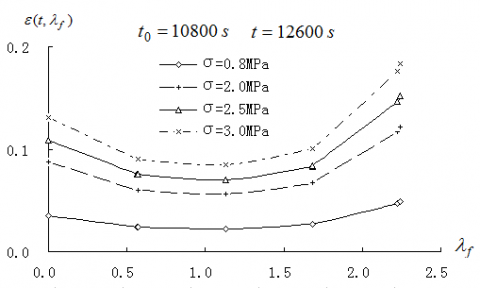## 9.png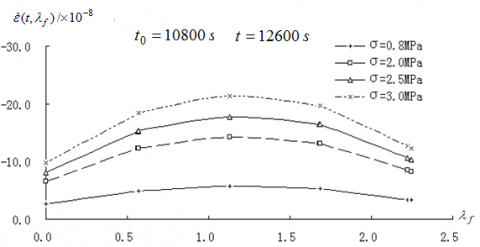By nonlinear fitting of our test data, the relationship between the model parameters of formulas (4) and (5) and the FCCP can be expressed as:

$\mathrm{E}_{1}\left(\lambda_{f}\right)=489.33+193.48 \lambda_{f}-97.46 \lambda_{f}^{2}$                (17)

$\mathrm{E}_{2}\left(\lambda_{f}\right)=110.91+59.49 \lambda_{f}-27.82 \lambda_{f}^{2}$               (18)

$\eta_{2}\left(\lambda_{f}\right)=327619+914046 \lambda_{f}-412653 \lambda_{f}^{2}$            (19)

$\mathrm{A}\left(\lambda_{f}\right)=36674.63+95928.89 \lambda_{f}-41400.4 \lambda_{f}^{2}$                   (20)

$\mathrm{B}\left(\lambda_{f}\right)=1.76 \times 10^{-4}+1.975 \times 10^{-3} \lambda_{f}-8.8 \times 10^{-4} \lambda_{f}^{2}$                 (21)

$\mathrm{E}_{2}\left(\lambda_{f}\right)=340.60+164.24 \lambda_{f}-72.98 \lambda_{f}^{2}$                (22)

${{\eta }_{2}}({{\lambda }_{f}})=108072.8+96344.6{{\lambda }_{f}}-41409.7\lambda _{f}^{2}$                   (23)

$\mathrm{A}\left(\lambda_{f}\right)=9729.09+18998.79 \lambda_{f}-7340.91 \lambda_{f}^{2}$                (24)

$\mathrm{B}\left(\lambda_{f}\right)=1.916 \times 10^{-3}+5.062 \times 10^{-3} \lambda_{f}-2.28 \times 10^{-3} \lambda_{f}^{2}$            (25)

Substituting formulas (17)-(21) and formulas (22)-(25) into formulas (4) and (5), respectively, the creep equation of the fiber-reinforced asphalt concrete can be established considering the influence of the FCCP:

$\varepsilon\left(t, \lambda_{f}\right)=\sigma_{0}\left[\frac{1}{\mathrm{E}_{1}\left(\lambda_{f}\right)}+\frac{1-e^{-B\left(\lambda_{f}\right) t}}{A\left(\lambda_{f}\right) B\left(\lambda_{f}\right)}+\frac{1-e^{-t \tau}}{\mathrm{E}_{2}\left(\lambda_{f}\right)}\right]$          (26)

$\varepsilon\left(t, \lambda_{f}\right)=\sigma_{0}\left[\frac{1-e^{-\mathrm{B}\left(\lambda_{f}\right) t_{0}}}{A\left(\lambda_{f}\right) B\left(\lambda_{f}\right)}+\frac{\left(1-e^{-t_{0} \tau}\right) e^{-\left(t-t_{0}\right) \tau\quad}}{\mathrm{E}_{2}\left(\lambda_{f}\right)}\right]$                   (27)

where, $\tau=E_{2}\left(\lambda_{f}\right) / \eta_{2}\left(\lambda_{f}\right)$.

Finding the derivative of formulas (26) and (27) relative to time t, respectively, the differential constitutive equation of the fiber-reinforced asphalt concrete can be established based on formulas (4) and (5) considering the influence of the FCCP:

$d\varepsilon (t,{{\lambda }_{f}})/dt=\dot{\varepsilon }(t,{{\lambda }_{f}})={{\sigma }_{0}}\left[ \frac{{{e}^{-B({{\lambda }_{f}})t}}}{A({{\lambda }_{f}})}+\frac{{{e}^{-t\tau }}}{{{\eta }_{2}}({{\lambda }_{f}})} \right]$                (28)

$d\varepsilon (t,{{\lambda }_{f}})/dt=\dot{\varepsilon }(t,{{\lambda }_{f}})={{\sigma }_{0}}\left[ \frac{({{e}^{-{{t}_{0}}\tau }}-1){{e}^{-(t-t{}_{0})\tau\quad }}}{{{\eta }_{2}}({{\lambda }_{f}})} \right]$           (29)

The creep equation and differential constitutive equation of the fiber-reinforced asphalt concrete derived from formula (4), and those derived from formula (2) can yield consistent results on the correlations of creep strain and creep rate with the FCCP, stress, and loading time. Similarly, The creep equation and differential constitutive equation derived from formula (5), and those derived from formula (3) can yield consistent results on the correlations of post-unloading creep strain and creep rate with the FCCP, stress, and loading time.

5. Conclusions

(1) With the growing volume ratio and aspect ratio of the fibers, the midspan deflection and bottom flexural-tensile strain of asphalt concrete beams first dropped and then rose over time. The lowest point of the time-creep deformation curve appeared at the fiber volume ratio of 0.348 and the fiber aspect ratio of 324. In this case, the fiber-reinforced asphalt concrete can resist rutting deformation excellently at the fiber volume ratio of 0.348 and the fiber aspect ratio of 324.

(2) The creep loading parameters of formulas (2) and (3) and the creep unloading parameters of formulas (4) and (5) all increased first and then decreased with the growth of fiber volume ratio and fiber aspect ratio. At the fiber volume ratio of 0.348 and the fiber aspect ratio of 324, the fiber-reinforced asphalt concrete is good at resisting elastic deformation, viscous flow deformation, and rutting deformation.

(3) The FCCP can reflect the overall effect of fiber volume ratio and fiber aspect ratio. Formulas (15) and (16) or formulas (28) and (29) are the viscoelastic constitutive equations of the fiber-reinforced asphalt concrete considering the influence of the FCCP. The greater the loading stress and the longer the loading time, the higher the creep strain and creep rate, the faster the recovery from creep deformation after unloading, and the larger the residual creep deformation. Creep strain, creep rate, and residual creep deformation after unloading all increased first and then decreased, with the growth of the FCCP. The optimal FCCP was 1.128 for polyester fibers.

Acknowledgment

The authors gratefully acknowledge the financial support of the project from the Colleges and Universities Key Scientific Research Projects of Henan Province (Grant No. 20A580006) and Horizontal Research Project of Xuchang University (Grant No. 2019HX005) and The Scientific Research Innovation Team of Xuchang University.

References

 Min, Z.H., Wang, X., Huang, W. (2004). Study on performance of epoxy asphalt concrete. Journal of Highway and Transportation Research and Development, 1(1): 1-4. 10.3969/j.issn.1002-0268.2004.01.001

 Lundström, R., Isacsson, U. (2004). Linear viscoelastic and fatigue characteristics of styrene–butadiene–styrene modified asphalt mixtures. Journal of Materials in Civil Engineering, 16(6): 629-638. https://doi.org/10.1061/(ASCE)0899-1561(2004)16:6(629)

 Polacco, G., Stastna, J., Biondi, D., Zanzotto, L. (2006). Relation between polymer architecture and nonlinear viscoelastic behavior of modified asphalts. Current Opinion in Colloid & Interface Science, 11(4): 230-245. https://doi.org/10.1016/j.cocis.2006.09.001

 Judycki, J. (1992). Non-linear viscoelastic behaviour of conventional and modified asphaltic concrete under creep. Materials and Structures, 25(2): 95-101. https://doi.org/10.1007/BF02472462

 Erkens, S.M.J.G., Liu, X., Scarpas, A. (2002). 3D finite element model for asphalt concrete response simulation. The International Journal Geomechanics, 2(3): 305-330. https://doi.org/10.1061/(ASCE)1532-3641(2002)2:3(305)

 Blab, R., Harvey, J.T. (2002). Modeling measured 3D tire contact stresses in a viscoelastic FE pavement model. The International Journal Geomechanics, 2(3): 271-290. https://doi.org/10.1061/(ASCE)1532-3641(2002)2:3(271)

 Yang, T.Q. (1990). Viscoelastic mechanics. Wuhan: Centra China University of Technology Press, pp. 8-28.

 Xu, S.F. (1992). A rheological model representing the deformation behavior of asphalt mixtures. Mechanics in Engineering, 14(1): 37-40.

 Ye, Y. (2009). The experience research of visco-elastoplastic constitute model of asphalt mixture. Wuhan: Solid Mechanics of Huazhong University of Science and Technology.

 Zhang, J.P., Xu, L., Wang, B. (2010). Modification of creep model of asphalt mixture and parameters determination. Journal of Wuhan University of Technology(Transportation Science & Engineering), 34(4): 699-702.

 Benedito, S.B., Wander, R.S., Dario, C.L. (2003). Engineering properties of fiber reinforced cold asphalt mixes. Jour-nal of Environmental Engineering, 129(10): 952-955. https://doi.org/10.1061/(ASCE)0733-9372(2003)129:10(952)

 Guo, N.S., Zhao, Y.H., Sun, L.L. (2004). Study on creep performance of fiber reinforced asphalt concrete. Journal of China & Foreign Highway, 1(1): 124-127. https://doi.org/10.3969/j.issn.1671-2579.2007.02.032

 Guo, N.S., Zhao, Y.H., Sun, L.L. (2007). Viscoelastic performance analysis of fiber reinforced asphalt concrete. Journal of Traffic and Transportation Engineering, 10(5): 37-40. https://doi.org/10.3321/j.issn:1671-1637.2007.05.009

 Feng, J.L. (2007). Studie of performance and mechanism of fiber reinforced asphalt mixtures. Nanjing: Department of Road and Railway Engineering of Dongnan University. https://doi.org/10.7666/d.y1040654

 JTJ 052-2000. (2000). Standard test methods of bitumen and bituminous mixtures for highway engineering. Beijing: China Communications Press.

 Chen, H.X., Zhang, Z.Q., Hu, C.S. (2004). Low-temperature anti-cracking performance of fiber-reinforced asphalt mixture. Huanan Ligong Daxue Xuebai(Ziran Kexue Ban)/ Journal of South China University of Technology(Natural Science Edition)(China), 32(4): 82-86. https://doi.org/https://doi.org/10.1007/BF02911033

 Szydlo, A., Mackiewicz, P. (2005). Asphalt mixes deformation sensitivity to change in rheological parameters. Journal of Materials in Civil Engineering, 17(1): 1-9. https://doi.org/10.1061/(ASCE)0899-1561(2005)17:1(1)

 Gao, D.Y., Liu, J.X. (1994). Basic theory for steel fiber reinforced concrete. Beijing: Science and Technology Literature Press, pp. 9-14.